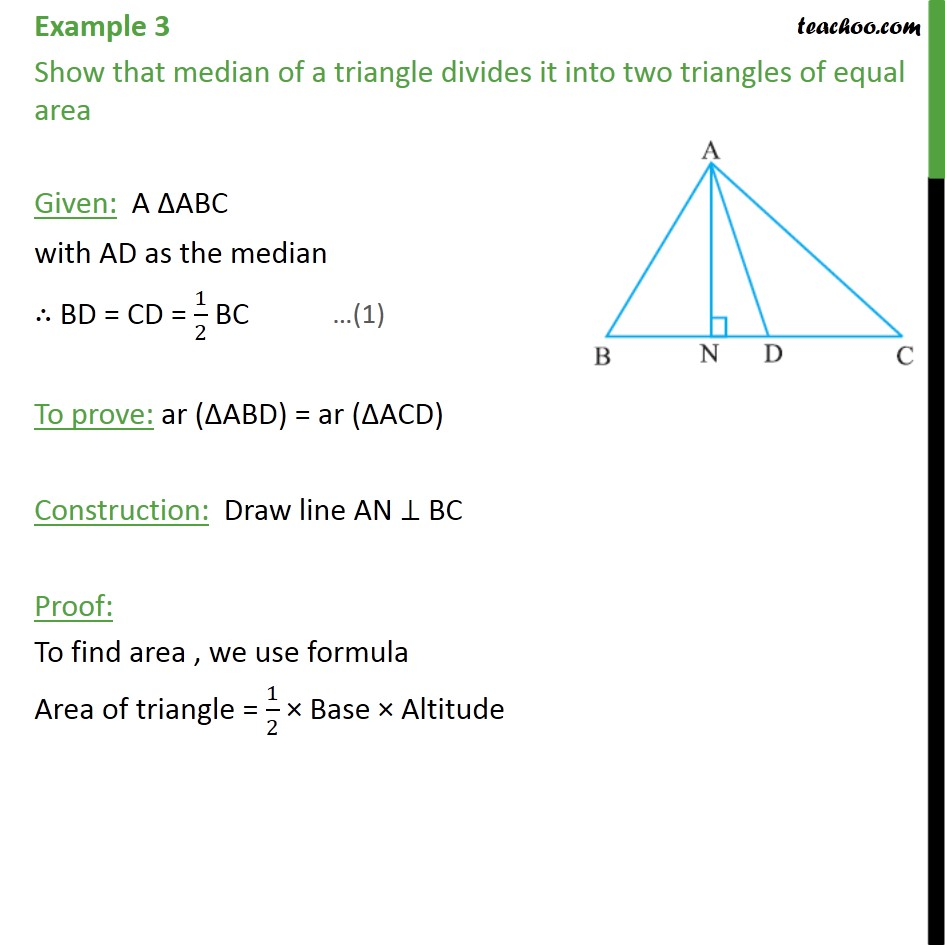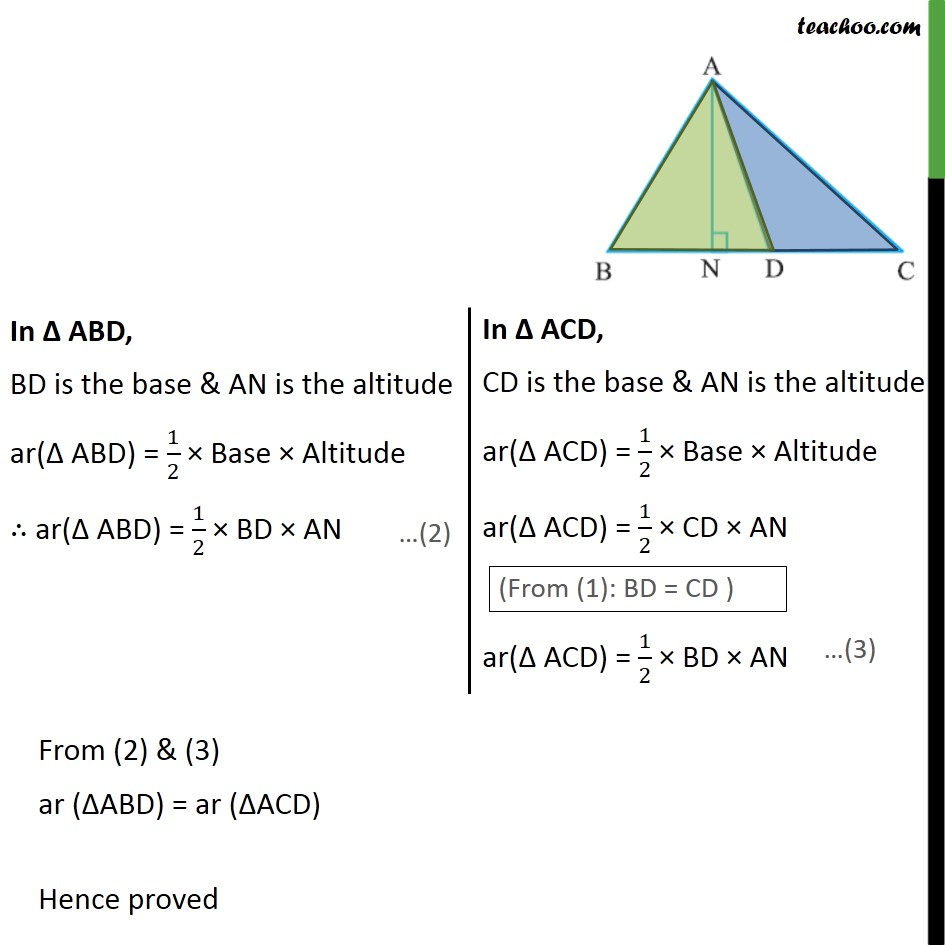Subscribe to our Youtube Channel - https://you.tube/teachoo

1. Chapter 9 Class 9 Areas of Parallelograms and Triangles
2. Concept wise
3. Median divides triangle into two triangles of equal area

Transcript

Example 3 Show that median of a triangle divides it into two triangles of equal area Given: A ABC with AD as the median BD = CD = 1/2 BC To prove: ar ( ABD) = ar ( ACD) Construction: Draw line AN BC Proof: To find area , we use formula Area of triangle = 1/2 Base Altitude From (2) & (3) ar ( ABD) = ar ( ACD) Hence proved

Median divides triangle into two triangles of equal area

About the AuthorDavneet Singh
Davneet Singh is a graduate from Indian Institute of Technology, Kanpur. He has been teaching from the past 9 years. He provides courses for Maths and Science at Teachoo.